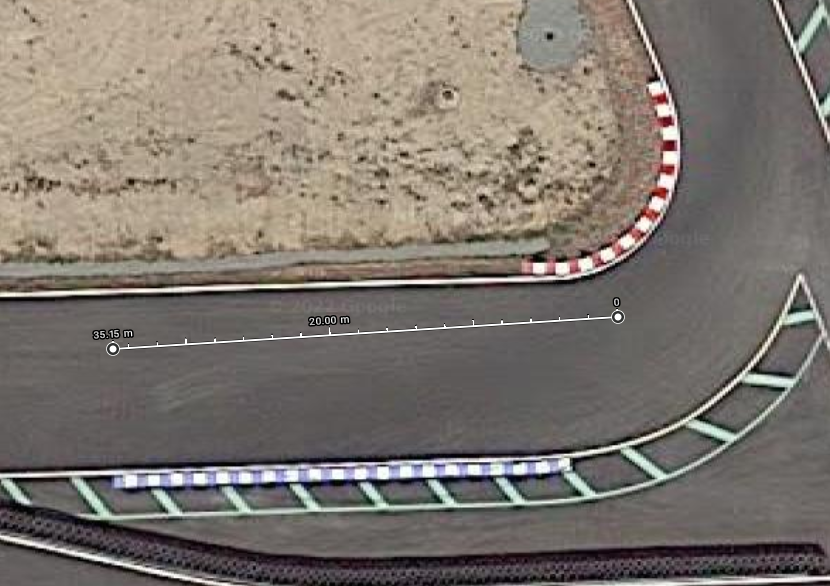## Kart Acceleration Calculator

(LO206)
Work In Progress - Please reachout to me if you have a suggestion or edit.

## Motivation

The purpose of this calculator is to determine how engine gearing, setup, weight, and initial speed effect the time required to travel straight line distance. Having a rough estimate of how weight and gearing affect a kart's forward acceleration provide useful insight.

## Method

I've created this calculator using the LO206 Briggs & Stratton data found here. The torque curve data has a small sample size, and a limited amount of data points. For this reason I linearly interpolated the LO206 torque curve to approximate values between undefined RPM ranges. I used euler integration to approximate the acceleration curve over time. I derived the equation for engine acceleration to be:

``````
a = (t * gr * 1.3358) / (m * wr)

where
a = acceleration (m/s^2)
t = torque (in ft-lbs)
gr = gear ratio = axle gear / clutch gear
m = mass (kg)
wr = wheel radius (m)

1.3358 is used to convert ft-lbs to newton meters
``````

## Limitations

Unfortunately, the calculator only provides an approximate output, and may not reflect real world performance. This is the consequence of a lack of data and precise calculation. First, the Briggs & Stratton data used for interpolating the engine torque curves only has a handful of data points. Second, the calculator does not account for altitude, air density, or other parameters which may affect engine performance. Third, this implementation of the calculator does not account for elevation changes. Fourth, the calculator does not handle linear resistance, although this may be negligible. Fifth, we do not account for tire grip, expansion, stretching, or any other parameters. In theory, we can account for all of these factors in future revisions of this calculator.

Although these factors may seem limiting, the calculator still provides useful insight on how a kart may accelerate.

If anyone has any data, knowledge, or found a significant inaccuracy, please do not hesitate to reachout to me.

## VIMC Analysis

I wanted to find how weight differences affect the time required to travel down the main straight at VIMC (Vancouver Island Motorsport Circuit) at race speeds. I found my initial speed coming on to the straight to be ~14 m/s. I found this number by observing video data and comparing it with a Google Maps view of the circuit.Using Google Maps I found VIMC to be about ~375 meters long.I used a gear ratio of 52 / 16, black carburetor slide, wheel radius of 0.1384m, and a drag coefficient of 0.1. The drag coefficient isn't based on any hard numbers or data. I used 0.1 because it matched my video data (it could be way off).

Results:
Kart weight of 163.293 kg takes 15.75 seconds to travel 375 meters.
Kart weight of 149.685 kg takes 15.59 seconds to travel 375 meters.

That is about a 0.16 second difference it takes to travel the main straight of VIMC.

Why does this happen? Because: Force = Mass * Acceleration.
Rearranged: Acceleration = Force / Mass.
Increase mass -> decrease acceleration -> heavier the kart, longer it takes to reach top speed.

This also means two karts traveling at the same top speed with different weight will travel the same amount of distance over a period of time. In this situation we can ignore drag, since we're limited by the RPM and gearing, not engine power and air resistance.

Note: We're not accounting for VIMC elevation changes.